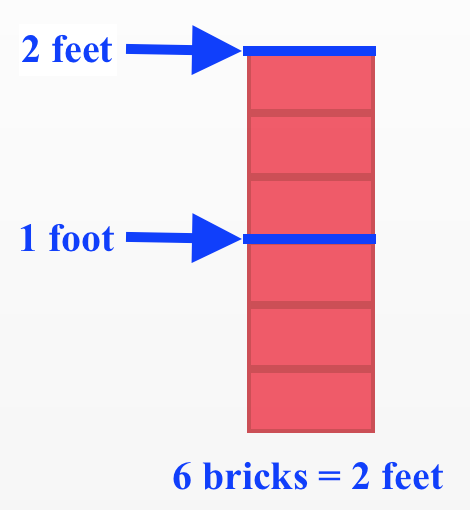### Home > CC2 > Chapter 4 > Lesson 4.2.4 > Problem4-66

4-66.

A stack of six bricks is two feet high. Use that information to answer the questions below. Homework Help ✎

1. How many bricks are in a stack $20$ feet high?

Use the diagram to the right for help.

($10$ two-foot stacks)($6$ bricks per two-foot stack) = $60$ bricks2. How high is a stack of $20$ bricks?

In part (a), you found that $60$ bricks make a stack $20$ feet high. Since $60=(3)(20)$, a stack of $20$ bricks should be one third the height of a stack of $60$ bricks.